# Inelastic Hinge Properties

Add, modify or delete inelastic hinge properties.

Inelastic hinges are applied to inelastic time history analysis only.

The Spring Type hinge defined in General Link Properties can be used for pushover analysis if the inelastic hinge properties are assigned to the hinge.

From the Main Menu Select Properties > Inelastic Properties > Inelastic Hinge > Inelastic Hinge Properties.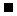Click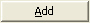to add Inelastic Hinge Properties.To modify previously defined Inelastic Hinge Properties, select a property name, click the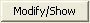button and change the input.To delete previously defined Inelastic Hinge Properties, select a property name and click the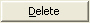button.To copy previously defined Inelastic Hinge Properties, select a property name and click the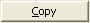button.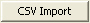: Import inelastic hinge properties saved in a CSV file.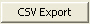: Export inelastic hinge properties to a CSV file. Only the inelastic hinge properties defined as User Type will be available for output. Only the inelastic hinge properties defined as Yield Strength (Surface) Calculation Method-User Type and Interaction Type-None will be available for output.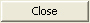: Close Inelastic Hinge Property dialog box.

####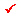Name

Enter the name representing the inelastic hinge properties.

####Description

Enter a brief description for inelastic hinge properties being defined.

####Yield Strength (Surface) Calculation Method

User Input: User directly defines inelastic hinge properties.

Auto-calculation: Inelastic hinge properties are automatically calculated using the selected material, section, and member information.

####Element Type

Beam-Column

Moment-Rotation(M-Theta): it concentrates the inelastic behavior represented by rotational and translational springs at each end and the center. And the remaining parts are assumed to behave elastically. Inelastic hysteresis behaviors are defined by skeleton curves, which are empirical hysteresis models. The axial component is represented by a spring at the center and two translational components are represented by springs at each end defined by force-displacement relationships. The two flexural components, My and Mz, are represented by springs defined by the relationship between moment and angle of rotation at either I or J or at both ends.

Moment-Curvature(M-Phi Distributed): Unlike lumped hinges, it assumes inelastic behavior throughout the member. The plastic hinge locations in the length direction of a member assigned by the user are defined as the integration points. The flexibility matrix of a section, which represents the distribution of internal forces, is calculated through the integration points. The number of integration points can be 1 and between 3 and 20. If the number of integration points is 2, the moment at the free end of a cantilever beam does not come to exactly zero due to the inherited characteristic of the integration method. Therefore, two integration points are not permitted. Inelastic hysteresis behavior can be defined by 2 models, empirical Skeleton and Fiber. The hinge behaviors can be expressed by force - deformation relationships in each axis direction, and the hinge hysteresis behavior of the flexural components can be expressed by the relationships of moment and angle of rotation. Inelastic behaviors can be defined for 3 axis components and 2 flexural (My & Mz) components.

Wall (CRB)

Inelastic properties of a CRB type wall element can be defined for 3 translational and 2 rotational directions. The inelastic hysteresis behavior of an element is defined by a hysteresis model.

General Link

Unlike Lumped and Distributed hinges, which are influenced by the inelastic properties of materials and members, the inelastic plastic hinge properties for the corresponding linear properties of each component of Property Type defined in General Link Properties are defined. The elastic stiffness of each component is defined by effective stiffness and acts as the initial stiffness in inelastic analysis. The inelastic hysteresis behavior of a spring is defined by a skeleton model. The inelastic properties of a spring can be defined for all 3 translational and 3 rotational directions. LRB and HDR type vibration isolator hysteresis models can be defined only by using Spring Type.

Note
In order to assign inelastic hinge properties to the Spring Type general link element that is defined in Model > Boundary > General Link Property, for pushover analysis, the Type must be set to "Spring"

Truss

The axial component is represented by a spring at the center defined by the force-displacement relationship. The inelastic hysteresis behavior of a spring is defined by a skeleton model.

####Material

Select a material type for the inelastic hinge properties to be applied. Depending on the type of selected material, the method of defining the yield stress of each element is evaluated differently.

Steel/SRC(filled): The 1st yielding is defined if the maximum flexural stress of a section reaches the yield stress. The 2nd yielding is defined if the flexural stress of the entire section reaches the yield stress.

RC/SRC(encased): The first yielding is defined if the maximum flexural stress of a section reaches the cracking stress of concrete. The second yielding is defined if the stress block of concrete reaches the ultimate strength or the rebars yield.

####Wall Type

Membrane: In-plane stiffness + rotational stiffness about the vertical direction. It is generally used to model shear walls being subjected to in-plane loads only.

Plate: in-plane stiffness + rotational stiffness about the vertical direct ion + out-of-plane bending stiffness. It is suitable for modeling common walls intended to resist in-plane loads as well as out-of-plane bending moments.

####Hinge Type

Define the hysteresis behavior model of an inelastic hinge.

Skeleton: It is an empirical hysteresis model, which assumes that the property of each directional component is independent of one another. Each component is defined as a uni-axial hinge hysteresis model. Such independently acting hinges are 3 translational and 3 rotational hinges.

Note
In the case where the inelastic hinge property is assigned to a Spring Type general link element for pushover analysis, the hysteresis model is automatically set to Skeleton.

Fiber: Fiber model is used to define multi-axis hinge hysteresis model.  In the fiber model, a beam section is divided into fibers, which undergo axial deformations only. By using the fiber model, the user can trace a more accurate moment-curvature relationship compared to using a hysteresis model of the member.  The moment-curvature relationship is based on the stress-strain relationship of the fiber material and an assumed strain distribution on the section.  Moreover, this model considers translation of neutral axis due to axial force.

####Interaction Type

For Column or Brace members, the type of considering interaction between axial force and moment is selected.

None: Interaction between axial force and moments is not considered.

Note
In the case where the inelastic hinge property is assigned to a Spring Type general link element for pushover analysis, the interaction between axial force and moment is automatically set to None.

P-M in Strength Calculation: N-M interaction in time history analysis is reflected by calculating the flexural yield strength of a hinge considering the effect of axial force. In this method, the interaction between biaxial bending moments is ignored. The axial force is assumed to act with each directional bending moment independently when the hinge status is evaluated at each time step. Recalculation of bending moment yield strength reflecting axial force is carried out in a loading condition, which satisfies the following three conditions:

1) It must be the first among the sequential time history load cases, which will be consecutively analyzed.

2) Inelastic static analysis must be carried out.

3) Displacement control must be used.

The elements are inelastic beam elements assigned with hinge properties to which P-M interaction is applied. The initial axial and bending moment at this time are determined by the combination of linear elastic analysis results of all the static loads contained in Time Varying Static Load. The factors used in the combination are defined by the Scale Factors specified in Time Varying Static Load.

P-M-M in Status Determination: This method uses a multi-axis hinge hysteresis model in inelastic time history analysis. Interaction between axial force and biaxial moments is realized by applying the plasticity theory. The interaction is considered at each time step through evaluating the status of inelastic hinges using the 3-dimensional yield surface. MIDAS/Civil supports the kinematic hardening type

Note
For Lumped and Distributed Types, the Fy and Fz components cannot consider interaction with axial force and moments
.

####Component Properties

Inelastic hinge properties by each component of section strength are entered.

Component

Select the components of sectional strength for which properties will be entered. The Spring Type permits properties in all directional components, whereas Lumped and Distributed types permit all but the Mx component.

Hinge Location

Select the locations of lumped inelastic hinges. Axial component is fixed to the center of a member. I-end, j-end or both ends can be selected for the bending moment components.

Num. of Sections

Enter the number of integration points for inelastic hinges of the distributed type. Up to 20 sections are permitted, and moment -curvature relationships are calculated at all the sections corresponding to the points.

Hysteresis Model

Select a hysteresis model for an inelastic hinge.

Properties

Enter the properties of inelastic hinges for each component.

Yield Surface Properties

If "P-M in Strength Calculation" or "P-M-M in Status Determination" is selected in Interaction Type, enter the related data for P-M interaction curve and 3-D yield surface.

Fiber Name

If a distributed hinge of the fiber type is selected, select a fiber element name.

####Hysteresis ModelKinematic hardening type hysteresis modelOrigin-oriented type hysteresis modelPeak-oriented type hysteresis modelClough type hysteresis modelDegrading Trilinear type hysteresis modelOriginal Takeda type hysteresis modelTakeda Tetra Linear  type hysteresis modelModified Takeda type hysteresis modelModified Takeda Tetra Linear type hysteresis modelNormal Bilinear type hysteresis modelElastic Bilinear type hysteresis modelElastic Trilinear type hysteresis modelElastic Tetralinear type hysteresis modelLRB Isolator Bilinear type hysteresis modelLRB Isolator Trilinear type hysteresis modelHigh Damping Rubber Isolator type hysteresis modelSlip Bilinear Type hysteresis modelSlip Trilinear Type hysteresis model

Note
When the General Link type is defined as inelastic hinge for inelastic time history analysis, all the hysteresis models provided by midas Gen can be used.

####Type

Select whether or not the skeleton curve is symmetrical. Non-symmetry of the curve can be applied to Yield Strength, Stiffness Reduction Ratio and Hinge Status. However, Kinematic Hardening Model does not permit non-symmetry of Stiffness Reduction Ratio due to its characteristics.

####Yield Properties

Input Method

User Input: User defines the Yield Properties.

Auto Calculation: The Yield Properties are automatically calculated.

Input Type

Strength-Stiffness Reduction Ratio: Yield Properties are defined by specifying Strength-Stiffness Reduction Ratio.

Strength-Yield Displacement: Yield Properties are defined by specifying Strength-Yield Displacement.

Yield Strength

Yield strength is specified. It is user defined based on material and section properties. The user specifies positive (+) values regardless of tension (t) or compression (c). The program treats compression as negative (-) internally.

P1: P1 represents the first yield strength. If the Material Type is Steel or SRC (filled), the first yield represents the state in which the maximum bending stress of the section reaches the yield stress. If the Material Type is RC or SRC (encased), the first yield represents the state in which the maximum bending stress of the section reaches the cracking stress of concrete.

P2: P2 represents the second yield strength. If the Material Type is Steel or SRC (filled), the second yield represents the state in which the bending stress of the entire section reaches the yield stress. If the Material Type is RC or SRC (encased), the second yield represents the state in which the stress in the concrete section reaches the ultimate strength or the stress in reinforcing steel reaches the yield strength. In case of bending, the concrete stress is based on a rectangular stress block.

P3: P3 represents the third yield strength.

Stiffness Reduction Ratio

Enter the stiffness reduction ratios of a sloped skeleton curve when Strength - Stiffness Reduction Ratio is selected for Input Type.

α1: Ratio of stiffness immediately after the first yielding divided by the initial stiffness

α2: Ratio of stiffness immediately after the second yielding divided by the initial stiffness, which is defined when the skeleton curve is of Trilinear or Tetralinear type.

α3: Ratio of stiffness immediately after the third yielding divided by the initial stiffness, which is defined when the skeleton curve is of Tetralinear type.

Yield Displacement

Enter the yield displacement of a sloped skeleton curve when Strength - Yield Displacement is selected for Input Type.

D1: first yield displacement component or deformation

D2: second yield displacement component or deformation, which is defined when the skeleton curve is of Trilinear or Tetralinear type.

D3: third yield displacement component or deformation, which is defined when the skeleton curve is of Tetralinear type.

####Deformation Indexes

Data required for calculating the indexes, which represent the level of deformation of an inelastic hinge

Ductility Factor: Select a basis of calculating ductility. Depending on the selection by the user, ductility factor is calculated by dividing the current deformation by the first yield deformation or the second yield deformation

Hinge Status: Enter the reference ductility, which classifies the state of a hinge in 5 different levels. In case of a non-symmetric hinge, the hinge status level at each time step is determined by the larger of the positive (+) and negative (-) levels. Level-1 (0.5) signifies the elastic status and Level-2 (1) signifies the yield status. Level-3 (2), Level-4 (4) and Level-5 (8) represent the level of ductility of each member. In analysis results, the status is presented in blue, green, yellowish light green, orange and red colors.

####Initial Stiffness

The initial stiffness used in inelastic analysis is either selected or entered by the user.

The selection of initial stiffness is founded on the premises of the longitudinal distribution of bending moment. If the bending moments, which are assumed to be linearly distributed, are the same in magnitude with opposite signs at both ends, select 6EI/L. If one end is 0, select 3EI/L. If the magnitudes and the signs are identical, select 2EI/L.

6EI/L , 3EI/L, 2EI/L: Provided that the inelastic hinge is of a lumped type for the bending moment component, the initial stiffness is selected on the basis of the longitudinal distribution of bending moment. This cannot be selected in case of Distributed Type and Spring Type.

6EI/L: when end values of linearly distributed bending moment are identical in magnitude but in opposite directions

3EI/L: when one end is 0

2EI/L: when the magnitudes and signs of end values are identical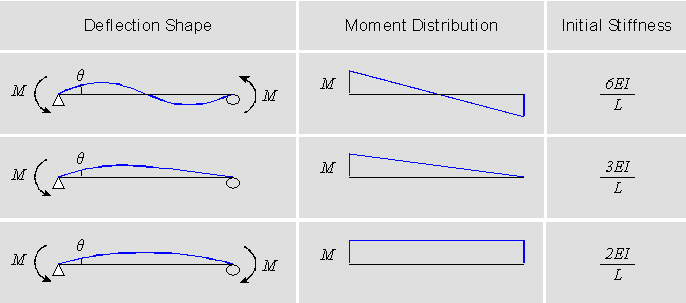User: the user directly enters the initial stiffness if the Input Type is Strength - Stiffness Reduction Ratio.

Elastic Stiffness: elastic stiffness of a member is used as the initial stiffness for inelastic analysis.

Skeleton Curve: when Strength - Yield Displacement is selected for the Input Type, the ratio of the user specified yield strength and yield displacement is used as the initial stiffness.

####Unloading Stiffness Parameter

Exponent in Unloading Stiffness Calculation: This is an option used to determine the unloading stiffness of the outer loop used in the Clough and Takeda type models among hysteresis models of skeleton curves. This is used to reflect the effect of reduction in stiffness, which occurs as the deformation progresses after yielding. The unloading stiffness is determined by the elastic stiffness reduced by the yield displacement and maximum displacement in the zone where unloading begins and the exponent entered here.

Inner Loop Unloading Stiffness Reduction Factor: This is used to determine the unloading stiffness of the inner loop. The inner loop is formed when unloading occurs before reaching the target point on the skeleton curve while reloading after the loading sign changes in the process of unloading. The unloading stiffness of the inner loop is calculated by multiplying the unloading stiffness of the outer loop by the reduction ratio for the unloading stiffness of the inner loop.

Yield Surface Properties

If "P-M in Strength Calculation" or "P-M-M in Status Determination" is selected in Interaction Type, enter the data related to P-M interaction curve and corresponding 3-dimentional yield surface.

####P-M Interaction Curves

Enter the P-M interaction curve data required to calculate 3-dimensional yield surfaces. All strength values must be entered with positive sign. Sign convention for plotting P-M curve is positive for compression and negative for tension.

Type of Input: Two input methods, user defined and auto-calculation based on material and section type, are supported to define the variables below. If some items are auto-calculated and the remainder is to be user defined, Auto-calculation should be performed first, and then necessary items can be modified after converting to User Input.

Crack Strengths
The following three items are required only if the Material Type is of RC or SRC (encased). All the numerical values are entered as positive.

NC0(t): Cracking strength due to pure tension force

MC0y: Cracking strength of a section subject to moment about y-axis without the presence of axial force

MC0z: Cracking strength of a section subject to moment about z-axis without the presence of axial force

The following 12 items are required irrespective of the Material Type. But for RC and SRC (encased) sections, approximate NC(t), NC(c), NCBy, NCBz, MCy,max and MCz,max are either entered or auto-calculated on the basis of NC0(t), MC0y and MC0z. All the numerical values are entered as positive.

Strengths for the 1st P-M Interaction Curves

NC(t): First yield strength subject to pure tension force

NC(c): First yield strength subject to pure compression force

NCBy: Axial force at the time of balanced failure in the first yield interaction curve for the y-axis moment of the section

NCBz: Axial force at the time of balanced failure in the first yield interaction curve for the z-axis moment of the section

MCy,max: Maximum bending yield strength in the first yield interaction curve for the y-axis moment of the section

MCz,max: Maximum bending yield strength in the first yield interaction curve for the z-axis moment of the section

Strengths for the 2nd P-M Interaction Curves

NY(t): Second yield strength subject to pure tension force

NY(c): Second yield strength subject to pure compression force

NYBy: Axial force at the time of balanced failure in the second stage yield interaction curve for the y-axis moment of the section

NYBz: Axial force at the time of balanced failure in the second yield interaction curve for the z-axis moment of the section

MYy,max: Maximum bending yield strength in the second yield interaction curve for the y-axis moment of the section

MYz,max: Maximum bending yield strength in the second yield interaction curve for the z-axis moment of the section

Shape of the 1st and 2nd P-M Interaction Curves:

Input the P-M interaction curve shapes. The shape of an interaction curve is defined by coordinates of 11 points among which the furthermost extreme coordinates for tension, compression and flexure are fixed to 0 or 1. Only the remaining 8 points are thus entered. If Material Type is RC or SRC (encased), the interaction curve for the first yield is of a linear shape and as such input is unnecessary. In calculating or displaying the axial component of the coordinates, the sign convention is (+) for compression and (-) for tension.

####Approximation of Yield Surface Shape

On the basis of P-M interaction curve, the parameters for 3-dimensional yield surface are either user defined or auto-calculated. If some items are auto-calculated and the remainder is to be user defined, Auto-calculation should be performed first, and then necessary items can be modified after converting to User Input. In case of Alpha, only user defined entry is possible. The value of each parameter is used in the equation of yield surface displayed in the dialog box.

Beta y, Beta z, Gamma: Being the exponential powers of P-My or P-Mz interactions, different values can be entered for the first and second yields. For Beta y and Beta z on the other hand, two separate values representing the ranges of larger and smaller axial forces relative to the axial force at the time of balanced failure can be entered. Alpha: Exponential powers of My-Mz interactions for the first and second yields.

Alpha: Exponent for My-Mz interaction for 1st and 2nd yielding

Coupling of Axial Force & Bending Moments: Select an interaction relationship between the components of axial and biaxial moments. Basically it is assumed that axial force and biaxial moments are inter-related in evaluating the hinge status. Nevertheless, the relationship between the axial force and the biaxial moments can be assumed to be either independent or coupled in formulating the stiffness matrix.

####Interaction Curves and Approximated Yield Surfaces

The P-M interaction curves entered by the user or calculated by material and section properties and the 3-dimensional yield surfaces composed from them are displayed. The yield surfaces are displayed by showing the outlines of projection on the reference plane. Through this can we then check how well the P-M interaction curves and the yield surfaces coincide.

Plot: Select an interaction curve or yield surface to be displayed. P-My, P-Mz or My-Mz can be selected.